## Sunday, April 3, 2011

### Dual Contouring

Dual Contouring is a method for meshing implicit surfaces, or surfaces which are defined as the level-set of scalar function. Conceptually it is similar to Marching Cubes, except that meshing is performed on a dual mesh, and requires that the scalar function be able to provide gradients or surface normals in addition to the function value.

The primary advantage of dual contouring is that it is able to reproduce sharp features, which is something that most other implicit surface meshing algorithms are unable to do. I also consider Dual Contouring to be simpler to implement from scratch than Marching Cubes, since you don't need to form large tables of stencils.

The overall algorithm is very simple. First the region to be meshed is divided into convex non-overlapping cells (like uniform cubes or tetrahedra). The scalar function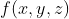that is being meshed is then evaluated at the vertices of those cells, and each vertex is labeled as being either inside or outside of the surface to be meshed.

Those cells that have a mix of inside and outside vertices must then contain a portion of the surface. It is this point where Dual Contouring begins to differ from Marching Cubes: instead of applying a stencil based on which vertices are inside or outside, Dual Contouring generates a single 'dual' vertex per cell straddling the surface. It then connects every dual vertex with it's neighboring dual vertices to form the final mesh. The only difficultly arises in choosing the location of the dual vertices to best represent the underlying surface being meshed.To choose where to place vertices, dual contouring uses not only the intersection of the surface with the edges of the mesh, but also the surface normals at those locations. It then solves for the dual vertex location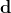which minimizes the error in satisfying planes that pass through the edge intersections with the same normals as at the intersections. This corresponds to minimizing the following error: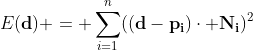whereis the dual vertex position,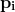is the location of the i'th (of n) edge intersection and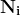is the corresponding normal for the i'th intersection. This is just a least squares system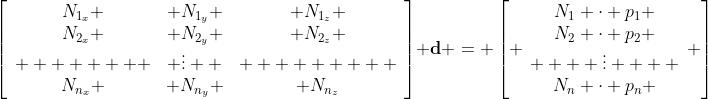which can be solved using a QR decomposition, or by forming the normal equations. One tricky aspect of this is that although there are always at least as many intersected edges as unknowns, it may not be the case that the row contributed by each edge is linearly independent from the other rows. In flat regions of the surface the normals will be nearly if not exactly the same, and the set of equations will be (nearly) singular.

One way to handle this is to add special-case code to check that the system is not singular, and if it is, solve a different system in 1D for the offset of the dual vertex in the normal direction that minimizes the fitting error. It is also possible to add a regularization term with low weight that prevents the system from being singular, but does not affect the results too badly in the event the system is well conditioned. I have done this by adding a (small) multiple of identity to the normal equations coefficient matrix, and the same small multiple of the original dual vertex position (i.e. the cell centroid) to the right hand side.

The image above shows an example of this in action. I generated an isosurface representation of the fandisk model, then ran it through dual contouring to produce the result you see above. Note that the method faithfully reproduces the sharp edges of this CAD model. I appear to have some minor bugs in the implementation however, as there are some artifacts on concave sharp edges.
The regularization approach seems to work well and eliminates the need for special case code. It also provides a knob to trade off mesh quality for approximation error: if the regularization term is small, then the method will try to reproduce the underlying surface faithfully. On the other hand, if the regularization term is large, dual vertices will be placed close to the centroid of the cells that generate them, producing a high quality mesh, but with staircasing artifacts where approximation error has been introduced.